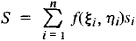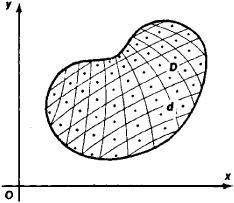# Multiple Integral

(redirected from Riemann multiple integral)

## multiple integral

[′məl·tə·pəl ′int·ə·grəl]
(mathematics)
An integral over a subset of n-dimensional space.

## Multiple Integral

an integral of a function defined on some region in a plane and in three-dimensional or n -dimensional space. The corresponding multiple integrals are referred to as double integrals, triple integrals, and n-tuple integrals, respectively.

Let the function f(x, y ) be defined on some region D of the plane xOy. Let us divide D into n subregions di whose areas are equal to si, choose a point (ξi, ηi) in each subregion di (see Figure 1), and form the integral sumIf as the maximal diameter of the subregions d, decreases without bound the sums S have a limit independent of the choice of the points (ξi, ηi), then this limit is called the double integral of the function f(x, y) over the region D and is denoted by

∫ ∫Df (x,y) ds

A triple integral and, in general, an n -tuple integral are defined analogously.Figure 1

In order for the double integral to exist, it is sufficient that, for example, the region D be a closed (Jordan) measurable region and that the function f(x, y) be continuous throughout D. Multiple integrals possess a number of properties similar to those of ordinary integrals. In order to calculate a multiple integral we reduce it to an iterated integral. Green’s formulas and the Green-Ostrogradskii theorem can be used in special cases to reduce multiple integrals to integrals of lower dimension. Multiple integrals find wide application. Volumes of bodies, as well as masses, static moments, and moments of inertia ( of bodies, for example) are expressed using multiple integrals.

Site: Follow: Share:
Open / Close